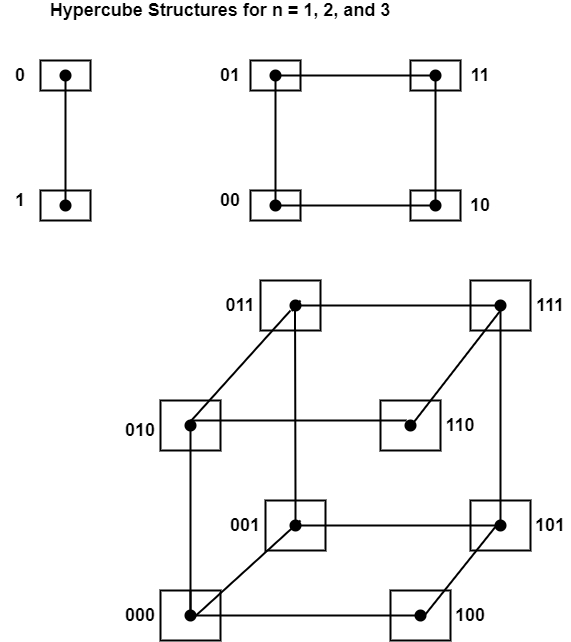# What is Hypercube Interconnection?

The hypercube interconnection is also defined as a binary n-cube multiprocessor. The hypercube is treated to be a loosely coupled system. This system is composed of N = 2n processors that are linked in an n-dimensional binary cube. Each processor denotes a node of the cube.

Each processor includes a direct communication route to n other neighbour processors. These routes are associated with the edges of the cube. The processors can be authorized with 2n distinct n-bit binary addresses. Each processor send differs from that of each of its n neighbors by directly one-bit position.

The figure displayed the hypercube structure for n, wherein n = 1, 2, and 3.A one-cube structure includes n = 1 and 2n = 2. It has two processors that are connected by an individual route. A two-cube structure includes n = 2 and 2n = 4. It has four nodes that are linked as a square. There are eight nodes associated as a cube in a three-cube structure.

There are 2n nodes in an n-cube structure with a processor actual in each node. A binary address is authorized to each node including the addresses of two neighbours vary in particularly one-bit position.

Routing messages through an n-cube structure can need one to n links, beginning from a source node to a destination node. For example, node 000 can interact directly with node 001 in a three-cube structure. It can communicate from node 000 to node 111, the message has to transit through a minimum of three links.

A routing phase can be developed by computing the exclusive-OR of the source node address with the destination node address. The resulting binary value will have 1 bit equivalent to the axes on which the two nodes differ. The message is then transmitted along with any one of the axes.

For example, in a three-cube structure, a message at 010 going to 001 makes an exclusive-OR of the two addresses similar to 011. The message can be transmitted along the second axis to 000 and then by the third axis to 001.

The description of the hypercube structure is the Intel iPSC computer complex. It consists of 128 (n = 7) microcomputers connected through communication channels. Each node includes a CPU, a floating-point processor, local memory, and serial communication interface units.

The individual nodes operate independently on data stored in local memory according to resident programs. The data and programs to each node come through a message-passing system from other nodes or a cube manager.